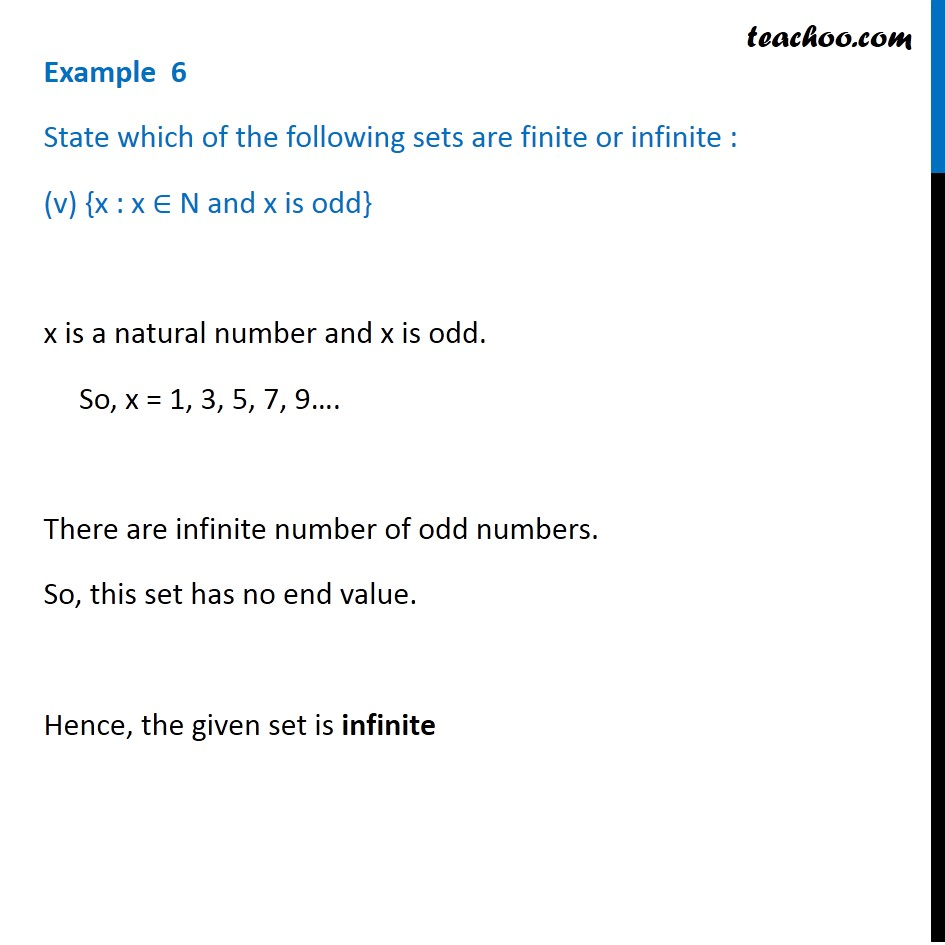Examples

Chapter 1 Class 11 Sets
Serial order wiseLearn in your speed, with individual attention - Teachoo Maths 1-on-1 Class

### Transcript

Example 6 State which of the following sets are finite or infinite : (v) {x : x ∈ N and x is odd} x is a natural number and x is odd. So, x = 1, 3, 5, 7, 9…. There are infinite number of odd numbers. So, this set has no end value. Hence, the given set is infinite